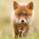216 views
216
EXPERIMENTAL: for evaluation.
Update: added candles/signal ma and options.
still didnt incorporate nyse adv/dec index's.
study(title="[RS]Modified McClellan Oscilator Candles V0")
//  ||----------------------------------------------------------------------------------------------------------------------------
//  source: http://stockcharts.com/school/doku.php?id=chart_school:technical_indicators:mcclellan_oscillator
//
//    McClellan Oscillator: 19-day EMA of RANA - 39-day EMA of RANA
//
//  19-day EMA* = (Current Day RANA - Prior Day EMA) * .10 + Prior Day EMA)
//  39-day EMA* = (Current Day RANA - Prior Day EMA) * .05 + Prior Day EMA)
//
//  * The first EMA calculation is a simple average.
//  ||----------------------------------------------------------------------------------------------------------------------------
fast_length = input(title='EMA - Fast Length:', type=integer, defval=19)
slow_length = input(title='EMA - Slow Length:', type=integer, defval=39)
USE_RANA = input(title='Use Ratio Adjustment Net Advancement:', type=bool, defval=true)
SHOW_SIGNAL = input(title='Show Lines:', type=bool, defval=true)
smooth_length = input(title='Smooth Signal Length:', type=integer, defval=4)

advance = cum(close > open ? close - open : 0)
decline = cum(close < open ? open - close : 0)

ma_fast = ema(adv_dec, fast_length)
ma_slow = ema(adv_dec, slow_length)

mcl_osc = ma_fast-ma_slow

signal_slow = sma(mcl_osc, smooth_length)
signal_slower = sma(mcl_osc, smooth_length*2)

palete = mcl_osc >= signal_slow ? lime : red
plotcandle(signal_slow, mcl_osc, mcl_osc, signal_slower, color=palete, wickcolor=gray)

plot(not SHOW_SIGNAL ? na : mcl_osc, color=mcl_osc>0?green:mcl_osc<0?maroon:gray, editable=true)
hline(0, color=black, editable=true)

signal = not SHOW_SIGNAL ? na : sma(mcl_osc, smooth_length)
plot(not SHOW_SIGNAL ? na : signal, color=black, linewidth=2, editable=true)fabfoot
the line can be customized on the options, arrows can be added later